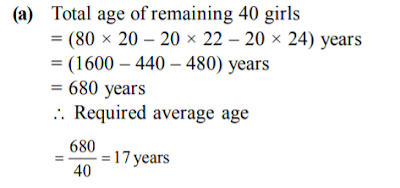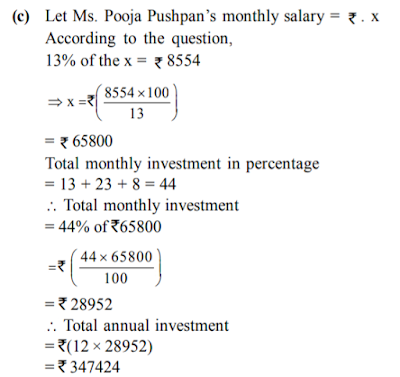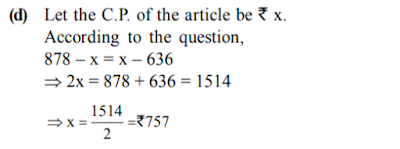# gkrecall

We brings you daily Current affairs, daily Current Affairs Quiz, weekly Current Affairs, weekly Current Affairs Quiz to enhance your preparation for upcoming exams. We also provide articles related to quant, reasoning, English, ssc etc .

## Breaking

We bring you the complete and important daily QUANT QUIZ to achieve more marks in Banking, Insurance, UPSC, SSC, CLAT, Railways and all other competitive Exams. We prepare it based on our daily current affairs.Hope you like it.

Web

START QUIZ

1. The simple interest accrued on an amount of `20,000 at
the end of three years is `7,200. What would be the
compound interest accrued on the same amount at the
same rate in the same period?
(a) ` 8098.56 (b) ` 8246.16
(c) ` 8112.86 (d) ` 8342.36
(e) None of these

2.If the numerator of a fraction is increased by 300% and the
denominator is increased by 500%, the resultant fraction
is 5/12 What was the original fraction?
(a)8/5
(b)5/11
(c)12/5
(d) 5/7
(e)none of these

3. A sum of money is divided among A, B, C and D in the
ratio of 3 : 5 : 9 : 13 respectively. If the share of C is 2412 more than the share of A, then what is the total
amount of money of B and D together?
(a) ` 4422 (b) ` 7236
(c) ` 6030 (d) ` 4824
(e) None of these

4. The average age of 80 girls was 20 years, the average age
of 20 of them was 22 years and that of another 20 was 24
years. Find the average age of the remaining girls.
(a) 17 years (b) 19 years
(c) 21 years (d) 15 years
(e) None of these

5. Ms. Pooja Pushpan invests 13% of her monthly salary, i.e.
`, 8554 in Mediclaim Policies, Later she invests 23% of her
monthly salary on Child. Education Policies; also she
invests another 8% of her monthly salary on Mutual
Funds. What is the total annual amount invested by Ms.
Pooja Pushpan?
(a) ` 28952 (b) ` 43428
(c) ` 347424 (d) ` 173712
(e) None of these

6. The profit earned after selling an article for `878 is the
same as loss incurred after selling the article for `636.
What is the cost price of the article?
(a) ` 797 (b) ` 787
(c) ` 767 (d) ` 757
(e) None of these

7. In a class of 240 students, each student got sweets got
sweets that are 15% of the total number of students.
How many sweets were there?
(a) 3000 (b) 3125
(b) 8640 (d) Cannot be determined
(e) None of these

8. Sonika spent `45, 760 on the interior decoration for her
home, `7896 on buying air conditioner and the remaining
28% of the total amount she had as cash with her. What
was the total amount?
(a) `98540 (b) `102300
(b) `134560 (d) Cannot be determined
(e) None of these

9. The ages of Khushi and Jagriti are in the ratio of 5 : 8
respectively. After 8 years the ratio of their ages will be
3 : 4 what is the difference in their ages?
(a) 16 years (b) 8 years
(c) 10 years (d) 12 years
(e) None of these

10. In how many different ways can the letters of the word
‘PUNCTUAL’ be arranged?
(a) 64 (b) 40320
(c) 960 (d) 20160
(e) None of these

DIRECTIONS (11-15) : What will come in place of the
question mark (?) in the following number series.

11. 93 95 99 ? 110 121 134
(a) 104 (b) 96
(c) 82 (d) 103
(e) None of these

12. 8 12 18 27 40.5 60.75?
(a) 104.125 (b) 121.125
(c) 96.125 (d) 83.125
(e) None of these

13. 4 7 11 18 29 ? 76 123
(a) 59 (b) 38
(c) 46 (d) 53
(e) None of these

14. 3 10 ? 172 885 5346 37471 299832
(a) 39 (b) 27
(c) 24 (d) 34
(e) None of these

15. 15 22 56 183 ? 3755 22542
(a) 709 (b) 698
(c) 748 (d) 800
(e) None of these

SOLUTION:

1.

2.

3.

4.5.6.7.8.9.10.

11. (d) The given number series is based on the following
pattern:
93 + 2 = 95
95 + 3 = 98
98 + 5 = 103
103 + 7 = 110
110 + 11 = 121
121 + 13 = 134
Hence, 103 will replace the question mark.

12. (e) The given number series is based on the following
pattern:
8 × 1.5 = 12
12 × 1.5 = 18
18 × 1.5 = 27
27 × 1.5 = 40.5
40.5 × 1.5 = 60.75
\ ? = 60.75 × 1.5 = 91.125
Hence, 91.125 will replace the question mark.

13. (e) The given number series is based on the following
pattern:
4 + 7 = 11
11 + 7 = 18
18 + 11 = 29
\ ? = 29 + 18 = 47
Hence, 47 will replace the question mark.

14. (a) The given number series is based on the following
pattern:
3 × 2 + 22
= 10
10 × 3 + 32
= 39
39 × 4 + 42
= 172
172 × 5 + 52
= 885
885 × 6 + 62
= 5346
Hence, 39 will replace the question mark.

15. (c) The given number series is based on the following
pattern :
15 × 1 + 1 × 7 = 22
22 × 2 + 2 × 6 = 56
56 × 3 + 3 × 5 = 183
183 × 4 + 4 × 4 = 748
748 × 5 + 5 × 3 = 3755
3755 × 6 + 6 × 2 = 22542
Hence, 748 will replace the question mark.